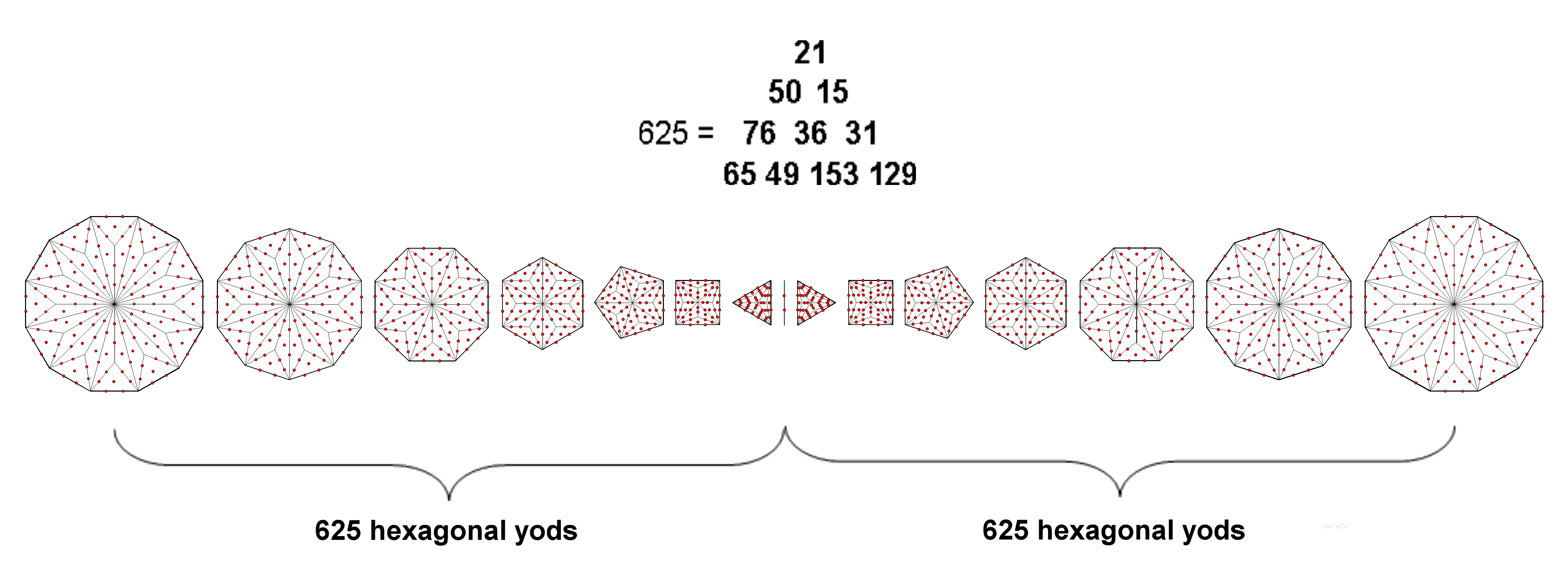<< Previous      2  3  4  5  ...36    Next >>

Miscellaneous

#1 The sum of the 10 Godname numbers is the number of hexagonal yods associated with a set of 7 separate, Type B polygons & the root edgeAn n-sided, Type B regular polygon has 13n hexagonal yods (see here). The seven separate regular polygons of the inner Tree of Life with 48 sides have (48×13=624) hexagonal yods. Consider the two sets of seven polygons separated by the root edge with its two hexagonal yods. One hexagonal yod in the root edge is associated with each set. 625 hexagonal yods are therefore associated with each set. This is the sum of the numbers of the Godnames of the 10 Sephiroth (listed here).

 << Previous      2  3  4  5  ...36    Next >>

Home